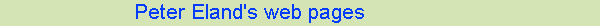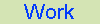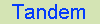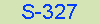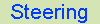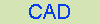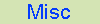# Tricycle steering geometry - FAQ

## How does the spreadsheet work?

What the spreadsheets do is express the geometry as co-ordinates (x, y). Each half of the linkage has two known points (where the linkage pivots on the frame) and two known lengths and angles for the steering arms (one on kingpin, one attached to handlebar) (which you enter).

Then, you can work out the length of the joining link using simple maths (Pythagoras) (with the steering straight ahead).

Then, if you turn the handlebars, you can compute the new position for the end of the lever attached to the bars.

The kingpin is still fixed... and you know the length of the lever attached to it, but not yet the angle. But you also know the length of the link joining it to the handlebar lever..

So you need to find a point which is a particular known radius from the kingpin, and another known radius from the end of the handlebar lever.

Make one fixed point (0,0) and the second (x1, y1), then by Pythagoras you can express the middle point in the linkage as an equation - which I don't have to hand - and each value (x, y) reduces to a quadratic form.

ax^2 + bx +c = 0

(I've lost my copy of the original workings, so if you work it out fully, please do send me a copy and I'll add it here!)

As it's just a quadratic equation, with the solution in the usual form:

x = (-b +- sqrt (b^2-4ac))/2a

Then you can choose whichever root looks sensible - this gives you the 'moving' link point, and you can then calculate the angle the wheels turn.

Do this both sides, compare with ideal geometry and it's done :-)

Previous: instructions -- Steering home -- Next: Archive